Solving Polynomial and Rational Inequalities

To solve an inequality such asor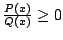where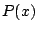and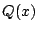are polynomials,

1. Factorandcompletely over the real numbers.

2. Mark the zeros ofandon a number line.

3. Determine the sign of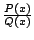on each of the resulting intervals.

4. Select the intervals corresponding to the sign of the original inequality. (If the inequality is not a strict inequality, include the zeros ofin the solution.)

In determining the sign ofon each interval, we can use the following:

Ifis the highest power of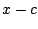which is a factor ofor, then

A. the sign ofchanges atifis odd; and

B. the sign ofdoes not change atifis even.
Ex 1 Solve the inequality.

Sol Factoring gives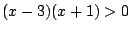; so marking off 3 and -1 on a number line and using the facts that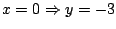and that the exponents of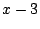andare both odd, we get the sign chart shown below: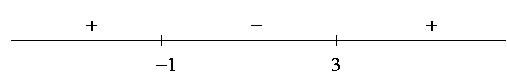Therefore the solution is given by.

Ex 2 Solve the inequality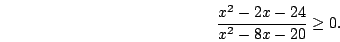Sol Factoring givesMarking off 6,-4,10, and -2 on a number line and using the facts thatand that the exponents of all the factors are odd, we get the sign chart shown below: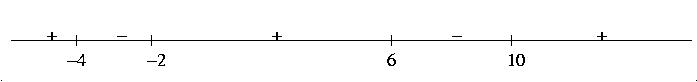Since the inequality is not strict, we can include the zeros of the numerator; so the solution is given by.

Pr 1 Solve the inequality.

Pr 2 Solve the inequality.

Pr 3 Solve the inequalityPr 4 Solve the inequalityPr 5 Solve the inequality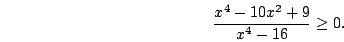Pr 6 Solve the inequalityPr 7 Solve the inequalityPr 8 Find all values offor which.

Pr 9 Find all values offor which